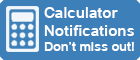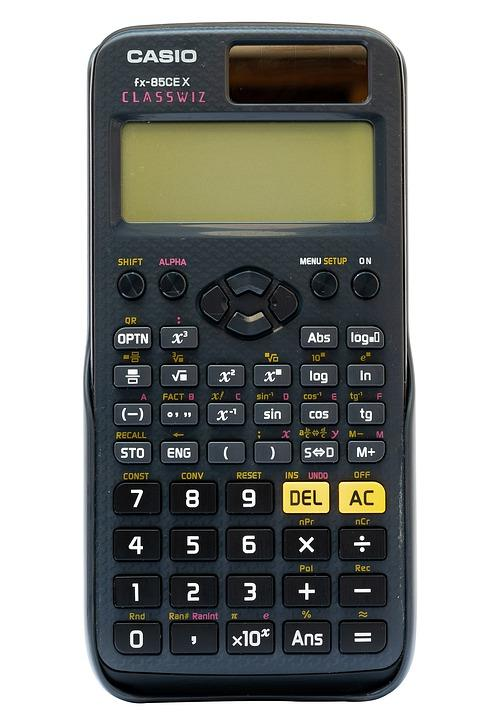Calculator Library
Free Online CalculatorsHome » 87 Calculators » Blog » How To Change A Calculator To Degrees?

Huge Domain Name Sale | Free Domains
Domain names on sale at crazy prices. Register a new domain for FREE when you buy another service.

## HOW TO CHANGE A CALCULATOR TO DEGREES?Do you need to convert between radians and degrees? Are you struggling to find the right button on your calculator? In this article, we'll show you how to change your calculator from radians to degrees. It's a very simple process, and with a little practice, you'll be able to do it in no time! We'll also provide a few tips that will help make the conversion process easier for you.Most people are more familiar with degrees than radians. A degree is a unit of measurement that's used to measure angles, and one complete circle is equal to 360 degrees. Radians, on the other hand, are a bit more complicated. A radian is defined as the ratio of the length of an arc to its radius.

One radian is equal to 57.2958 degrees, which means that there are approximately six radians in one complete circle. So, how do you know which unit of measurement to use? In most cases, it doesn't matter which one you use. However, there are some situations where it's more convenient to use radians. For example, when working with trigonometric functions, it's often easier to use radians.

It's better to convert between the two units before you start working on a problem. This way, you can avoid any confusion and make sure that you're using the right unit of measurement.

## Degree Mode Vs. Radian Mode On A Calculator

The degree mode on a calculator is simply another way of measuring angles. When you're in radian mode, your calculator will measure angles in terms of radians. However, when you're in degree mode, your calculator will measure angles in terms of degrees. The degree mode is often denoted by the symbol “°”.

## How To Change Calculator To Degrees?

First of all, test what mode your calculator is currently in by trying to calculate the value of sin 90. If the answer is 1, then your calculator is currently in degree mode. If the answer is 0.89399, then your calculator is currently in radian mode.

To change from radian mode to degree mode, simply press the "MODE" button on your calculator. This will bring up a menu with different settings that you can change. Use the arrow keys to navigate to the "DEG" setting and press "ENTER". Your calculator should now be in degree mode. To change from degree mode to radian mode, simply repeat the process and select the "RAD" setting instead.

The step by step process to change your calculator from radians to degrees is given as follows:

1. Locate the "Mode" button on your calculator. This button is usually located near the top of the calculator.
2. Press the "Mode" button until you see the word "DEG" on the display. This indicates that your calculator is now in degree mode.
3. Use the calculator as normal.

On some calculator models, such as the Casio FX-5500L and others, you might need to press the "Mode" button twice or more. On calculators like the FX-83GT Plus, you may have to press "Shift" and "Mode" to display the appropriate menu screen. If none of these methods work, consult your model's handbook to discover the button combination that works for your specific model.

## Converting Between Radians and Degrees

If you need to convert between radians and degrees, there's a simple formula that you can use. To convert from radians to degrees, multiply the number of radians by 57.2958. To convert from degrees to radians, divide the number of degrees by 57.2958. For example, if you want to convert pi radians to degrees, you would multiply pi by 57.2958, which would give you 180 degrees.

Other examples include:

• Convert 45 degrees to radians: 45 divided by 57.2958 equals 0.7854 radians.
• Convert 0.523598775 radians to degrees: 0.523598775 multiplied by 57.2958 equals 30 degrees.
• Convert pi/12 radians to degrees: pi/12 multiplied by 57.2958 equals 15 degrees.
• Convert 60 degrees to radians: 60 divided by 57.2958 equals.

## How To Change Calculator To Degrees On An Online Calculator?

Online calculators come with a wide variety of features and settings. Most online calculators come with direct options to change the mode from radians to degrees. For example, on the online calculators, you can simply click on the "Deg" button to change the mode from radians to degrees.

## Other Features In A Scientific Calculator

A scientific calculator comes with a wide variety of features and settings. In addition to having the ability to change the mode from radians to degrees, most scientific calculators also come with other features, such as:

• Calculate the value of sin, cos, tan and other trigonometric functions.
• Calculate the value of logarithms.
• Calculate the value of exponential functions.
• Solve equations.
• Graph functions.
• Convert between different units of measurement.
• Perform statistical calculations.
• Calculate the value of permutations and combinations.
• Calculate the value of factorials.
• And much more!

If you're having trouble changing the mode on your online calculator, consult the calculator's user manual for instructions. Most online calculators have a "Help" or "FAQ" section that can be accessed from the calculator's main menu.

## Conclusion

In conclusion, changing the mode on your calculator from radians to degrees is a relatively simple process. Most calculators have a "Mode" button that can be used to change the mode.

Remember, when working with angles, it's often more convenient to use radians. However, in some cases, you may need to use degrees. Knowing how to change the mode on your calculator will allow you to work with whichever unit of measurement is more appropriate for the task at hand.

 Previous Post« What Does RPN Mean On A Calculator? Next PostHow To Do Fractions On A Calculator? »